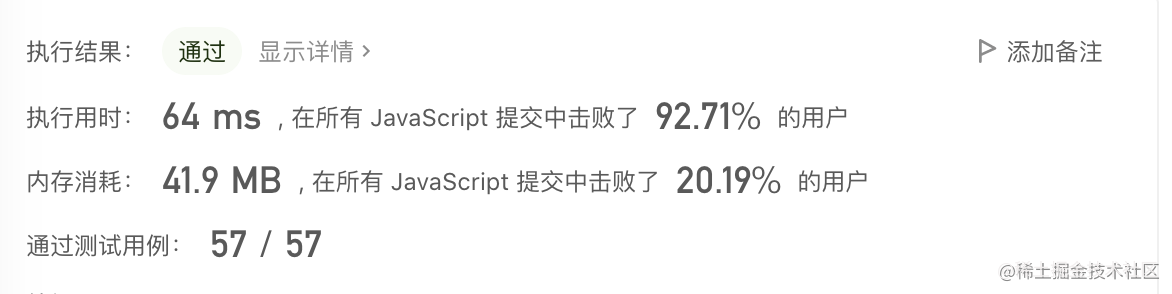# 蚂蚁金服笔试真题：如何防止重复请求？两数之和求解?## 如何防止重复发送请求？

``````// 示例
let count = 1;
let promiseFunction = () =>
new Promise(rs =>
window.setTimeout(() => {
rs(count++);
})
);
let firstFn = firstPromise(promiseFunction);
firstFn().then(console.log); // 1
firstFn().then(console.log); // 1
firstFn().then(console.log); // 1

### 题目解析

``````
function firstPromise(promiseFunction) {
let p = null;
return function (...args) {
// 请求的实例，已存在意味着正在请求中，直接返回实例，不触发新的请求
return p
? p
// 否则发送请求，且在finally时将p置空，那么下一次请求可以重新发起
: (p = promiseFunction.apply(this, args).finally(() => (p = null)));
};
}

``````let count = 1;
let promiseFunction = () =>
new Promise((rs) =>
setTimeout(() => {
rs(count++);
}, 1000)
);
let firstFn = firstPromise(promiseFunction);
firstFn().then(console.log); // 1
firstFn().then(console.log); // 1
firstFn().then(console.log); // 1

setTimeout(() => {
firstFn().then(console.log); // 2
firstFn().then(console.log); // 2
firstFn().then(console.log); // 2
}, 3000);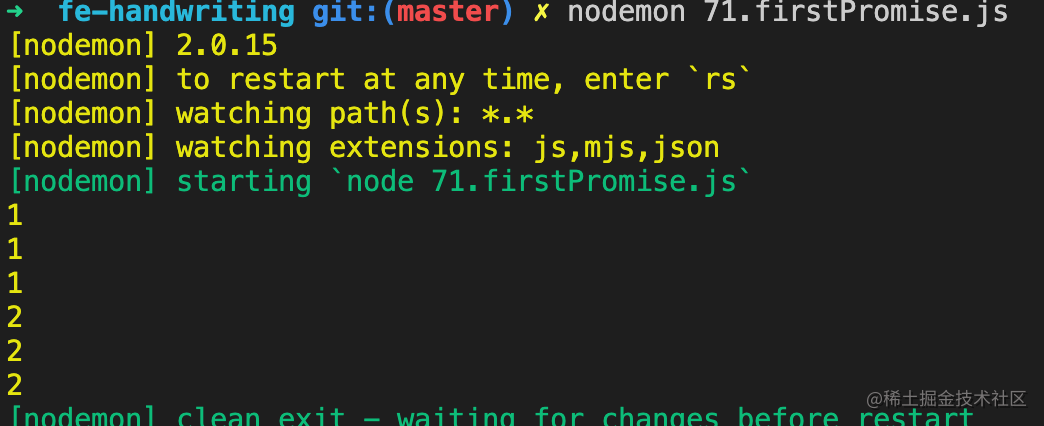## 两数之和

``````示例 1：

2 <= nums.length <= 104
-109 <= nums[i] <= 109
-109 <= target <= 109

### "憨厚"直觉法

``````const twoSum = (nums, target) => {
const len = nums.length
for (let i = 0; i < len; i++) {
// 同一个元素在答案里不能重复出现所以j = i + 1
for (let j = i + 1; j < len ; j++) {
// 找到答案，return
if (nums[ i] + nums[ j ] === target) {
return [ i, j ]
}
}
}
}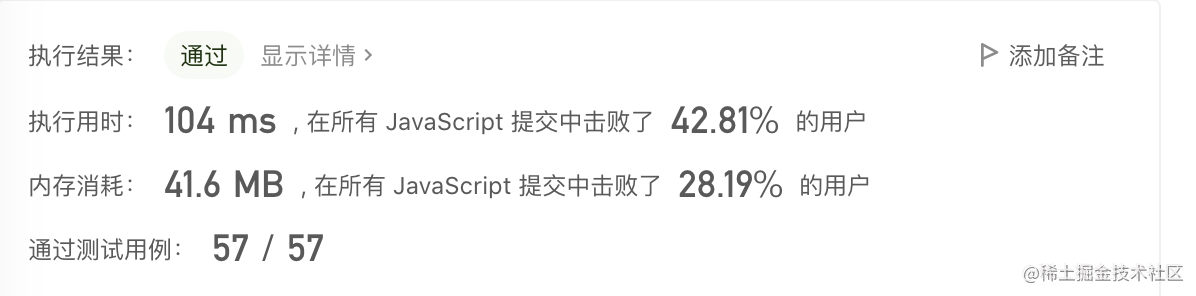`进阶：你可以想出一个时间复杂度小于 O(n^2) 的算法吗？`，题目的最后提醒我们有没有时间复杂度小于O(n^2)的算法，就是说还有更优解。

### "Map"空间换时间法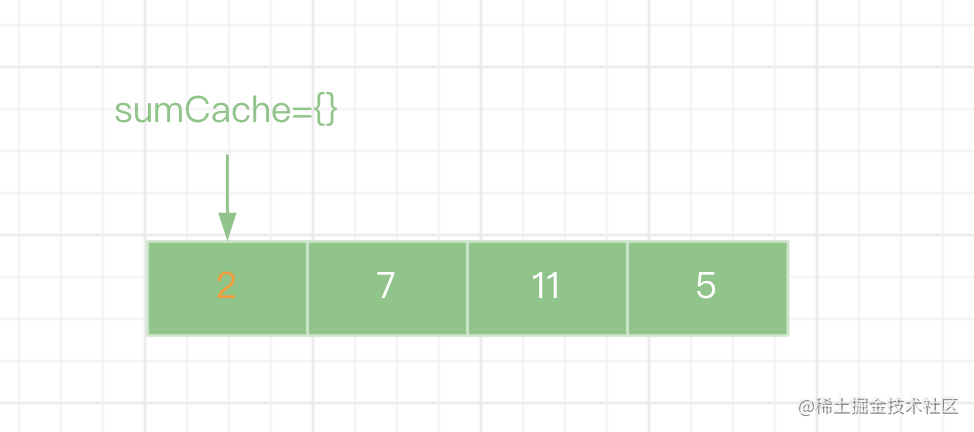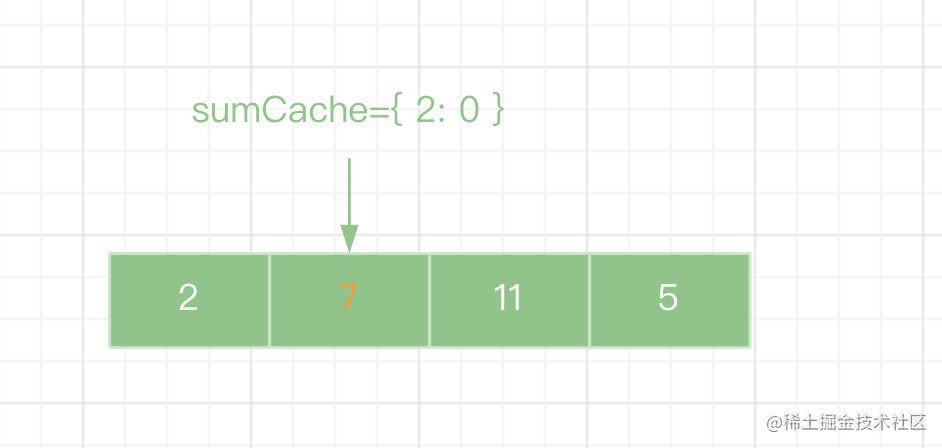``````const twoSum = (nums, target) => {
const len = nums.length
const sumCache = {}

for (let i = 0; i < len; i++) {
const value = nums[ i ]
// 计算差值
const diff = target - value
// 如果差值已经存在过，直接返回对应的索引
if (typeof sumCache[ diff ] !== 'undefined') {
return [ sumCache[ diff ], i ]
} else {
// 否则存起来
sumCache[ value ] = i
}
}
}